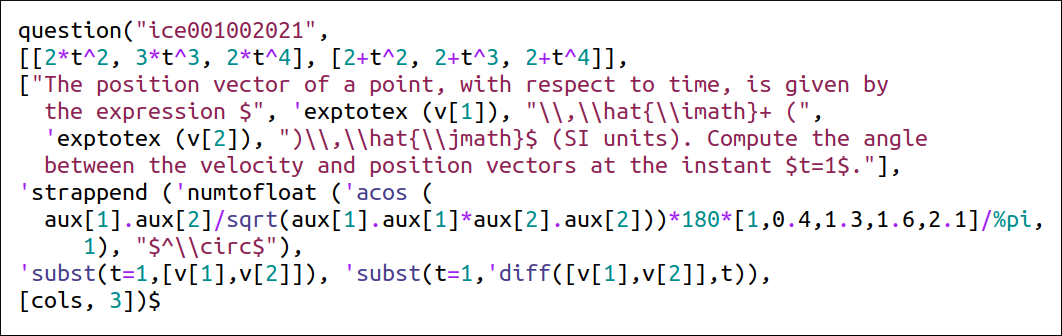# Question generation with Maxima

I have written a Maxima program, quiz.mac, to help create the exams.

It has a function question that inserts questions into a dictionary. The structure of that function is:

question ("name", [param1,param2,..], "question text", answers, [auxiliary_expressions...], [options...])

The following example shows a question which is solved as in the Maxima example given in the previous slide.A value for the variable v is chosen from the list of 3 expressions [2*t^2, 2*t^3, 2*t^4] and a value for v is chosen from the list [2+t^2, 2+t^3, 2+t^4].

The results of the two commands in the second to last line are saved into the auxiliary variables aux and aux.